#### Chapter 20 Surface Area and Volume of a Right Circular Cone R.D. Sharma Solutions for Class 9th Exercise 20.2

Exercise 20.2

1. Find the volume of a right circular cone with:
(i) Radius 6 cm, height 7 cm.
(ii) Radius 3.5 cm, height 12 cm
(iii) Height 21 cm and slant height 28 cm.

Solution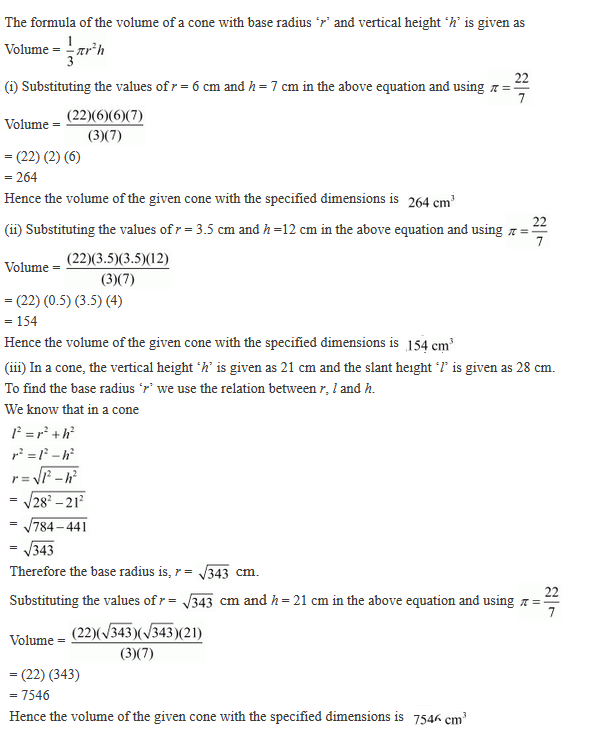2.  Find the capacity in litres of a conical vessel with:
(i) radius 7 cm, slant height 25 cm
(ii) height 12 cm, slant height 13 cm.

Solution3. Two cones have their heights in the ratio 1 : 3 and the radii of their bases in the ratio 3 : 1. Find the ratio of their volumes.

Solution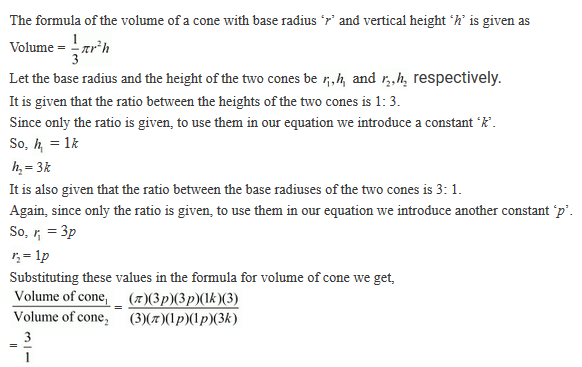4. The radius and the height of a right circular cone are in the ratio 5 : 12. If its volume is 314 cubic metre, find the slant height and the radius .  (Use π = 3.14) .

Solution5. The radius and height of a right circular cone are in the ratio 5 : 12 and its volume is 2512 cubic cm. Find the slant height and radius of the cone.
(Use π = 3.14).

Solution6. The ratio of volumes of two cones is 4 : 5 and the ratio of the radii of their bases is 2 : 3, Find the ratio of their vertical heights.

Solution7. A cylinder and a cone have equal radii of their bases and equal heights. Show that their volumes are in the ratio 3 : 1.

Solution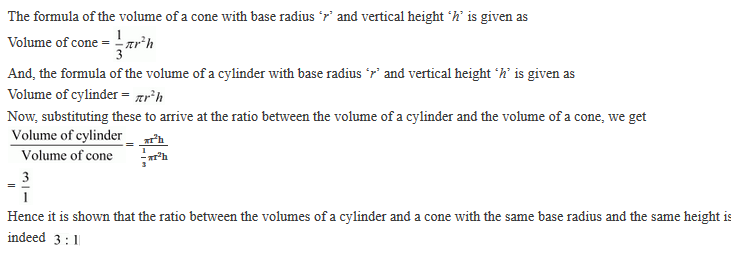8. If the radius of the base of a cone is halved, keeping the height same, what is the ratio of the volume of the reduced cone to that of the original cone ?

Solution9.  A heap of wheat is in the form of a cone of diameter 9 m and height 3.5 m. Find its volume. How much canvas cloth is required to just cover the heap? (Use π = 3.14).

Solution10. Find the weight of a solid cone whose base is of diameter 14 cm and vertical height 51 cm, supposing the material of which it is made weighs 10 grams per cubic cm.

Solution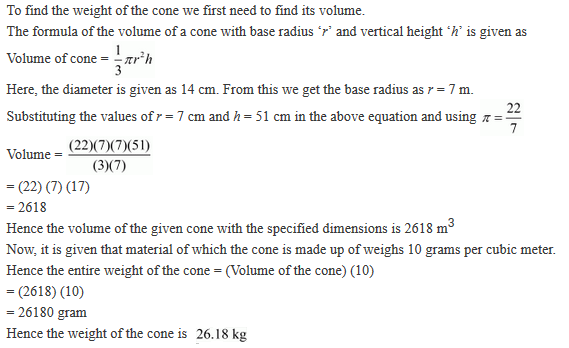11.  A right angled triangle of which the sides containing the right angle are 6.3 cm and 10 cm in length, is made to turn round on the longer side. Find the volume of the solid, thus generated. Also, find its curved surface area.

Solution12. Find the volume of the largest right circular cone that can be fitted in a cube whose edge is 14 cm.

Solution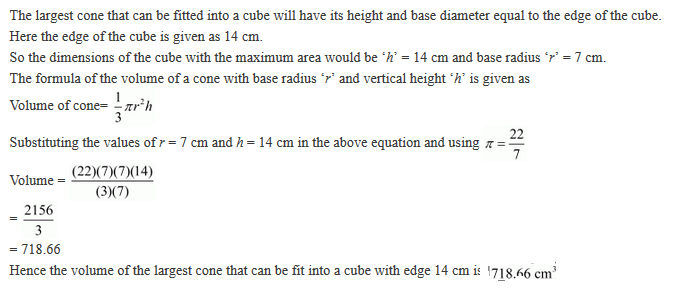13. The volume of a right circular cone is 9856 cm3. If the diameter of the base is 28 cm, find:
(i) height of the cone
(ii) slant height of the cone
(iii) curved surface area of the cone.

Solution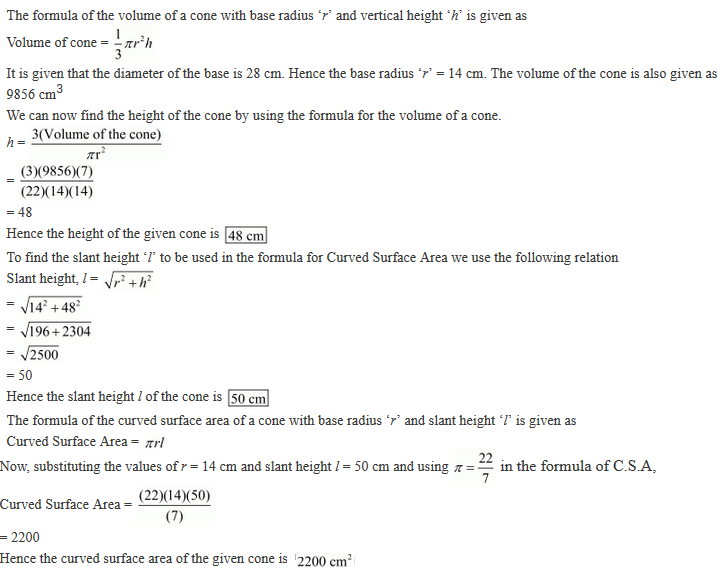14. A conical pit of top diameter 3.5 m is 12 m deep. What is its capacity in kilolitres?

Solution15. Monica has a piece of Canvas whose area is 551 m2. She uses it to have a conical tent made, with a base radius of 7 m. Assuming that all the stitching margins and wastage incurred while cutting, amounts to approximately 1 m2. Find the volume of the tent that can be made with it.

Solution